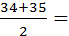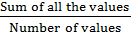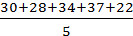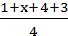# Paper 2 Project Maths Essential Revision Notes1. Averages 3

2. Frequency Distribution Tables 8

3. Bar Charts and Pie Charts 20

4. Stem and Leaf Plot 26

5. Probability 31

6. Points, Lines and Angles and Triangles 39

7. Circles 47

8. Triangles inside circles 53

9. Congruent and similar Triangles 59

10. Trigonometry 66

11. Constructions 76

12. Co-ordinate Geometry of the line 85

13. Transformations 98

14. Cylinder and Spheres 102

15. Pyramids and Cones 113

1. Averages

The mode is the value that occurs most often.

The mode of 20,22,25,26,28,22,25,22,28,20,26,22 is 22 as it occurs the most amount of times in the sequence.

The median is the middle value when the values are arranged in order of size.

To get the median of 14,21,17,11,19,15,16 we must first rearrange our values in ascending order

11,14,15,16,17,19,21

Our middle value of the sequence is 16 so this is our median

If we are getting the median of an even number of values we must get the average of our middle two values

To get the median of 34, 36, 30, 28, 42, 38, 33, 35 we first arrange in ascending order

28, 30, 33, 34, 35, 36, 38, 42

Our middle values are 34 and 35 so our median is 34.5The mean of a set of values is the sum of all the values divided by the number of values.

Mean =To get the mean of 30, 28, 34, 37, 22 we add up all our values and divide by the number of values which in this case is 5

Mean = = 30.2The range or spread of a set of values is the difference between the highest value and the lowest value

The mean, mode and median are sometimes called the measures of central tendency

Questions

1. The mean of 5 numbers is 12 find the sum of the numbers

Mean =Mean × Number of values = Sum of all the values

5 × 12 = 60

2. In 4 games, a soccer player scored 1, x, 4 and 3 goals respectively. The mean number of goals scored by the player per game was 2.

Find the number of goals scored in the second game i.e. the value of x.

Mean == 28 + x = (4)(2)

8 + x = 8

x = 0

3. Given the numbers 42,44,39,52,37,44,49,42,44,40 Find the mode, median and mean.

Mode ...